# 从PyTorch到PyTorch Lightning —一个简要介绍

【导读】这篇文章回答了关于PyTorch Lightning最常见的问题。

PyTorch很容易使用，可以用来构建复杂的AI模型。但是一旦研究变得复杂，并且将诸如多GPU训练，16位精度和TPU训练之类的东西混在一起，用户很可能会写出有bug的代码。

PyTorch Lightning完全解决了这个问题。Lightning会构建您的PyTorch代码，以便抽象出训练的详细信息。这使得AI研究可扩展并且可以快速迭代。

PyTorch Lightning适合谁使用？

PyTorch Lightning是NYU和FAIR在进行博士研究时创建的

「PyTorch Lightning是为从事AI研究的专业研究人员和博士生所创建的」。

Lightning是在我攻读纽约大学CILVR的人工智能研究和Facebook的AI研究的博士学位中诞生的。该框架被设计为具有极强的可扩展性，同时又使最先进的AI研究技术（例如TPU训练）变得很简单。

Lightning拥有超过96名贡献者，由8名研究科学家，博士研究生和专业深度学习工程师组成的核心团队。

• 模型
• 数据
• 损失
• 优化器

# 模型

3层网络（由William Falcon所创建）

Lightning为PyTorch代码提供结构看到了吗？这两个代码完全相同！或将其用作预训练模型

# 数据

1. 下载图片。
2. 图像变换（这是由个人而定的）。
3. 拆分成训练集，验证集和测试集。
4. 将每个拆分后的数据集包装在DataLoader中。

「prepare_data」

「train_dataloader, val_dataloader, test_dataloader」

Lightning甚至允许对于测试集或验证集创建多个数据加载器。

# 优化器

Lightning「可扩展性很强」。例如，如果您想使用多个优化器（例如、GAN），则可以在此处返回两个优化器。

# 损失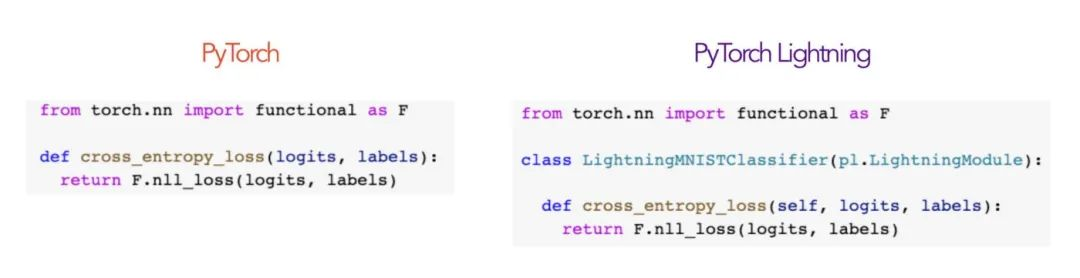再次强调……代码是完全一样的！

1. 模型（3层的神经网络）
2. 数据集（MNIST）
3. 优化器
4. 损失

• 迭代多个epoch（一个epoch是对数据集「D」的完整遍历）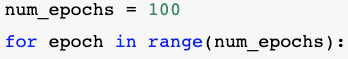在代码中

• 每个epoch迭代批处理大小为「b」的小块数据集• 我们执行前向传播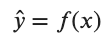在数学上在代码中

• 计算损失在代码中

• 执行后向传播以计算每个权重的所有梯度

• 将梯度应用于每个权重

Lightning的训练器(Trainer)

PyTorch完整的训练循环的代码

```import torch
from torch import nn
import pytorch_lightning as pl
from torch.utils.data import DataLoader, random_split
from torch.nn import functional as F
from torchvision.datasets import MNIST
from torchvision import datasets, transforms
import os
# -----------------
# 模型
# -----------------
class LightningMNISTClassifier(pl.LightningModule):
def __init__(self):
super(LightningMNISTClassifier, self).__init__()
# MNIST 图片 (1, 28, 28) (channels, width, height)
self.layer_1 = torch.nn.Linear(28 * 28, 128)
self.layer_2 = torch.nn.Linear(128, 256)
self.layer_3 = torch.nn.Linear(256, 10)
def forward(self, x):
batch_size, channels, width, height = x.sizes()
# (b, 1, 28, 28) -> (b, 1*28*28)
x = x.view(batch_size, -1)
# 第1层
x = self.layer_1(x)
x = torch.relu(x)
# 第2层
x = self.layer_2(x)
x = torch.relu(x)
# 第3层
x = self.layer_3(x)
# 标签的概率分布
x = torch.log_softmax(x, dim=1)
return x
# ----------------
# 数据
# ----------------
transform=transforms.Compose([transforms.ToTensor(), transforms.Normalize((0.1307,), (0.3081,))])
mnist_train = MNIST(os.getcwd(), train=True, download=True, transform=transform)
mnist_test = MNIST(os.getcwd(), train=False, download=True, transform=transform)
# 训练集 (55,000 images), 测试 (5,000 images)
mnist_train, mnist_val = random_split(mnist_train, [55000, 5000])
mnist_test = MNIST(os.getcwd(), train=False, download=True)
# 数据加载器进行shuffling, batching等等的操作。。。
mnist_train = DataLoader(mnist_train, batch_size=64)
mnist_val = DataLoader(mnist_val, batch_size=64)
mnist_test = DataLoader(mnist_test, batch_size=64)
# ----------------
# 优化器
# ----------------
pytorch_model = MNISTClassifier()
optimizer = torch.optim.Adam(pytorch_model.parameters(), lr=1e-3)
# ----------------
# 损失
# ----------------
def cross_entropy_loss(logits, labels):
return F.nll_loss(logits, labels)
# ----------------
# 训练循环的代码
# ----------------
num_epochs = 1
for epoch in range(num_epochs):
# 训练循环
for train_batch in mnist_train:
x, y = train_batch
logits = pytorch_model(x)
loss = cross_entropy_loss(logits, y)
print('train loss: ', loss.item())
loss.backward()
optimizer.step()
optimizer.zero_grad()
# 验证循环
with torch.no_grad():
val_loss = []
for val_batch in mnist_val:
x, y = val_batch
logits = pytorch_model(x)
val_loss.append(cross_entropy_loss(logits, y).item())
val_loss = torch.mean(torch.tensor(val_loss))
print('val_loss: ', val_loss.item())```

Lightning完整的训练循环的代码

lightning代码与PyTorch完全相同，除了：

• 核心成分由LightningModule进行组织
• 训练/验证循环的代码已由培训器(trainer)抽象化
```import torch
from torch import nn
import pytorch_lightning as pl
from torch.utils.data import DataLoader, random_split
from torch.nn import functional as F
from torchvision.datasets import MNIST
from torchvision import datasets, transforms
import os
class LightningMNISTClassifier(pl.LightningModule):
def __init__(self):
super(LightningMNISTClassifier, self).__init__()
# MNIST 图片 (1, 28, 28) (channels, width, height)
self.layer_1 = torch.nn.Linear(28 * 28, 128)
self.layer_2 = torch.nn.Linear(128, 256)
self.layer_3 = torch.nn.Linear(256, 10)
def forward(self, x):
batch_size, channels, width, height = x.size()
# (b, 1, 28, 28) -> (b, 1*28*28)
x = x.view(batch_size, -1)
# 第1层 (b, 1*28*28) -> (b, 128)
x = self.layer_1(x)
x = torch.relu(x)
# 第2层 (b, 128) -> (b, 256)
x = self.layer_2(x)
x = torch.relu(x)
# 第3层 (b, 256) -> (b, 10)
x = self.layer_3(x)
# 标签的概率分布
x = torch.log_softmax(x, dim=1)
return x
def cross_entropy_loss(self, logits, labels):
return F.nll_loss(logits, labels)
def training_step(self, train_batch, batch_idx):
x, y = train_batch
logits = self.forward(x)
loss = self.cross_entropy_loss(logits, y)
logs = {'train_loss': loss}
return {'loss': loss, 'log': logs}
def validation_step(self, val_batch, batch_idx):
x, y = val_batch
logits = self.forward(x)
loss = self.cross_entropy_loss(logits, y)
return {'val_loss': loss}
def validation_epoch_end(self, outputs):
# 在验证结束时调用
# 输出是一个数组，包含在每个batch在验证步骤中返回的结果
# 输出 = [{'loss': batch_0_loss}, {'loss': batch_1_loss}, ..., {'loss': batch_n_loss}]
avg_loss = torch.stack([x['val_loss'] for x in outputs]).mean()
tensorboard_logs = {'val_loss': avg_loss}
return {'avg_val_loss': avg_loss, 'log': tensorboard_logs}
def prepare_data(self):
# 图像变换对象
transform=transforms.Compose([transforms.ToTensor(),
transforms.Normalize((0.1307,), (0.3081,))])

# 对MNIST进行变换
mnist_train = MNIST(os.getcwd(), train=True, download=True, transform=transform)
mnist_test = MNIST(os.getcwd(), train=False, download=True, transform=transform)

self.mnist_train, self.mnist_val = random_split(mnist_train, [55000, 5000])
def train_dataloader(self):
return DataLoader(self.mnist_train, batch_size=64)
def val_dataloader(self):
return DataLoader(self.mnist_val, batch_size=64)
def test_dataloader(self):
return DataLoader(self,mnist_test, batch_size=64)
def configure_optimizers(self):
optimizer = torch.optim.Adam(self.parameters(), lr=1e-3)
return optimizer
# 训练
model = LightningMNISTClassifier()
trainer = pl.Trainer()
trainer.fit(model)```

1. 如果没有Lightning，则可以将PyTorch代码分为任意部分。使用Lightning之后，代码是结构化的。
2. 除了在Lightning中进行结构化之外，这两者的代码完全相同。（这值得大笑两声）。
3. 随着项目的复杂性增加，您的代码将不用改变其中的大部分的代码内容。
4. 保留了PyTorch的灵活性，因为您可以完全控制训练中的关键点。例如，您可以使用任意复杂的training_step，比如seq2seq
```def training_step(self, batch, batch_idx):
x, y = batch

# 定义您的前向传播和损失函数
hidden_states = self.encoder(x)

# 甚至像seq-2-seq+attn模型一样复杂
# （这只是一个示例代码，用来进行说明的）
start_token = '<SOS>'
last_hidden = torch.zeros(...)
loss = 0
for step in range(max_seq_len):
attn_context = self.attention_nn(hidden_states, start_token)
pred = self.decoder(start_token, attn_context, last_hidden)
last_hidden = pred
pred = self.predict_nn(pred)
loss += self.loss(last_hidden, y[step])

#示例代码
loss = loss / max_seq_len
return {'loss': loss}```
1. 在Lightning中，您可以使用很多附加的功能，例如进度条

tensorboard日志（是的！您什么都没有做）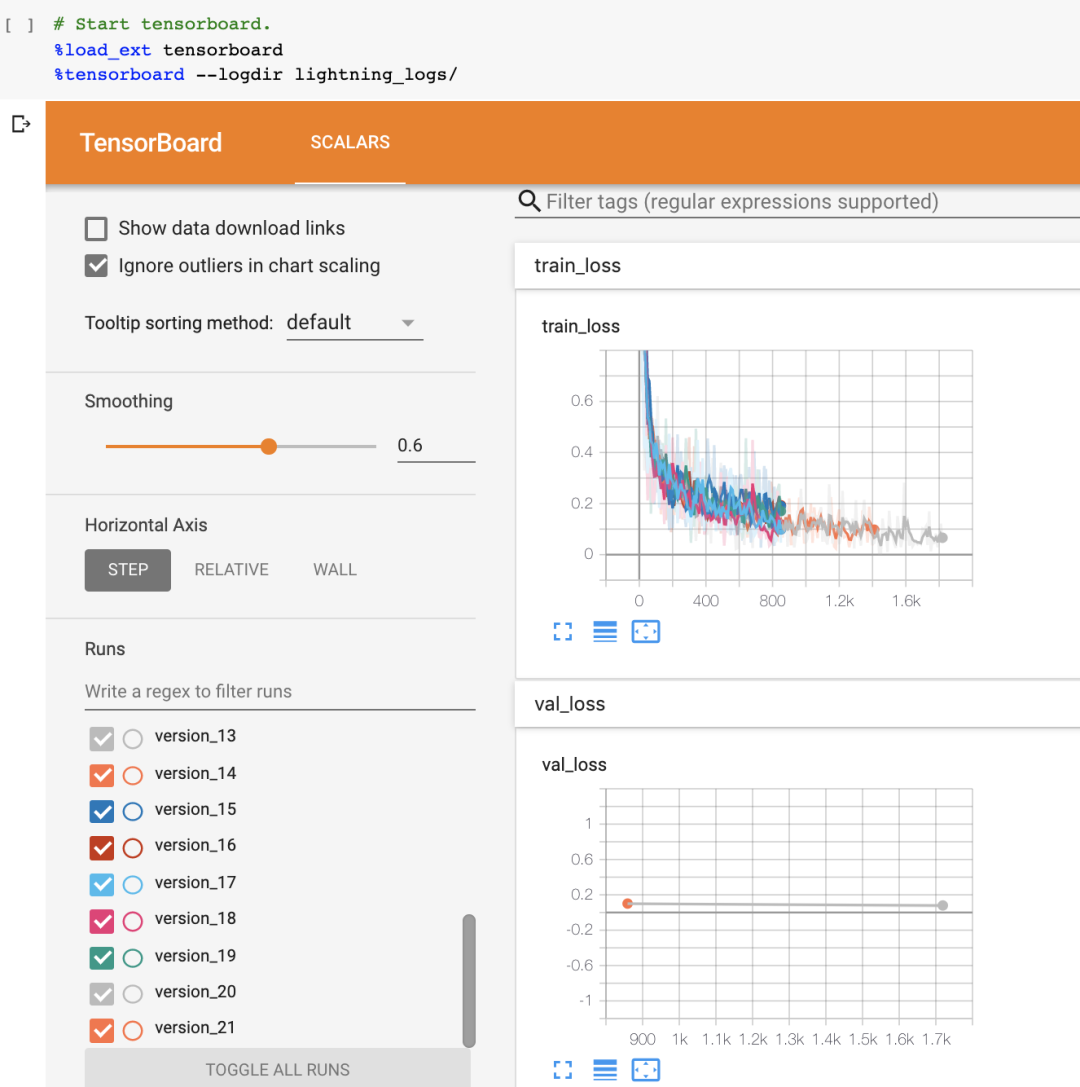Lightning是以开箱即用（例如TPU训练等）而闻名的。

• 前向传播
• 后向传播
• 应用优化器
• 进行分布式训练
• 设置16位精度
• 如何截断后向传播
• 您需要配置的任何内容

这些覆盖发生在LightningModule中

可扩展性的回调

回调是您希望在训练的各个部分中执行的一段代码。在Lightning中，回调用于非必需的代码，例如日志记录或与研究代码无关的东西。这样可以使研究代码保持超级干净和有条理。

假设您想在训练的各个部分进行打印或保存一些内容。这是回调的样子

现在，您将其传递给培训器，该代码将在任意时间被调用

这种方法将您的研究代码组织在三个不同的存储桶(buckets)中

1. 研究代码（LightningModule）（这是科学）。
2. 工程代码（培训器）
3. 与研究无关的代码（回调）

如何开始

希望本指南向您确切地介绍了Lightning如何入门。最简单的开始方法是运行带有MNIST示例的colab笔记本。

或安装Lightning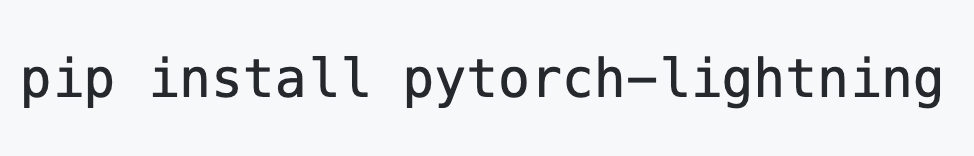或查看Github页面。

原文链接：

来自 “ ITPUB博客 ” ，链接：http://blog.itpub.net/31555081/viewspace-2698296/，如需转载，请注明出处，否则将追究法律责任。

全部评论

注册时间：2018-09-17

• 博文量
53
• 访问量
68637